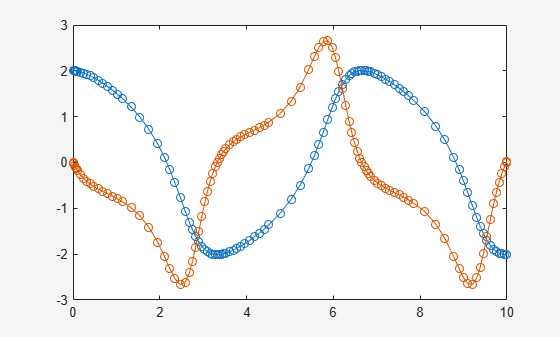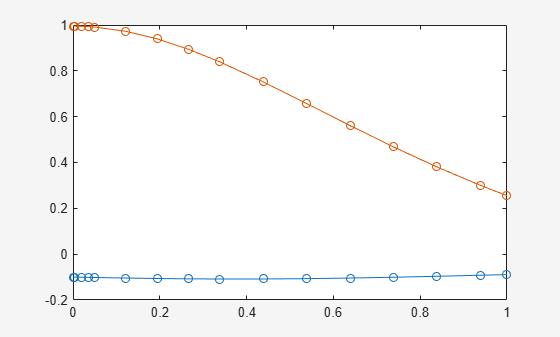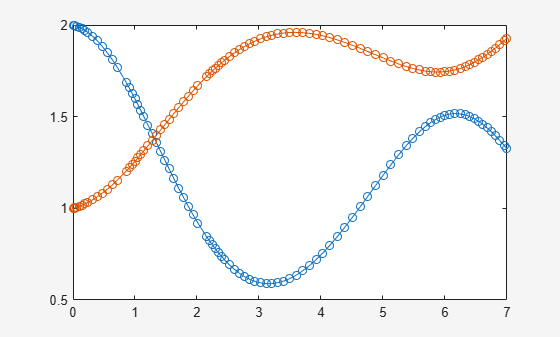# odeFunction

Convert symbolic expressions to function handle for ODE solvers

## Syntax

``f = odeFunction(expr,vars)``
``f = odeFunction(expr,vars,p1,...,pN)``
``f = odeFunction(___,Name,Value)``

## Description

example

````f = odeFunction(expr,vars)` converts a system of symbolic algebraic expressions to a MATLAB® function handle. This function handle can be used as input to the numerical MATLAB ODE solvers, except for `ode15i`. The argument `vars` specifies the state variables of the system.```

example

````f = odeFunction(expr,vars,p1,...,pN)` specifies the symbolic parameters of the system as `p1,...,pN`.```

example

````f = odeFunction(___,Name,Value)` uses additional options specified by one or more `Name,Value` pair arguments.```

## Examples

collapse all

Convert a system of symbolic differential algebraic equations to a function handle suitable for the MATLAB ODE solvers. Then solve the system by using the `ode15s` solver.

Create the following second-order differential algebraic equation.

```syms y(t); eqn = diff(y(t),t,2) == (1-y(t)^2)*diff(y(t),t) - y(t);```

Use `reduceDifferentialOrder` to rewrite that equation as a system of two first-order differential equations. Here, `vars` is a vector of state variables of the system. The new variable `Dy(t)` represents the first derivative of `y(t)` with respect to `t`.

`[eqs,vars] = reduceDifferentialOrder(eqn,y(t))`
```eqs = diff(Dyt(t), t) + y(t) + Dyt(t)*(y(t)^2 - 1) Dyt(t) - diff(y(t), t) vars = y(t) Dyt(t)```

Set initial conditions for `y(t)` and its derivative `Dy(t)` to `2` and `0` respectively.

`initConditions = [2 0];`

Find the mass matrix `M` of the system and the right sides of the equations `F`.

`[M,F] = massMatrixForm(eqs,vars)`
```M = [ 0, 1] [ -1, 0] F = - y(t) - Dyt(t)*(y(t)^2 - 1) -Dyt(t)```

`M` and `F` refer to the form $M\left(t,x\left(t\right)\right)\stackrel{˙}{x}\left(t\right)=F\left(t,x\left(t\right)\right).$. To simplify further computations, rewrite the system in the form $\stackrel{˙}{x}\left(t\right)=f\left(t,x\left(t\right)\right)$.

`f = M\F`
```f = Dyt(t) - Dyt(t)*y(t)^2 - y(t) + Dyt(t)```

Convert `f` to a MATLAB function handle by using `odeFunction`. The resulting function handle is input to the MATLAB ODE solver `ode15s`.

```odefun = odeFunction(f,vars); ode15s(odefun, [0 10], initConditions)```Convert a system of symbolic differential equations containing both state variables and symbolic parameters to a function handle suitable for the MATLAB ODE solvers.

Create the system of differential algebraic equations. Here, the symbolic functions `x1(t)` and `x2(t)` represent the state variables of the system. The system also contains constant symbolic parameters `a`, `b`, and the parameter function `r(t)`. These parameters do not represent state variables. Specify the equations and state variables as two symbolic vectors: equations as a vector of symbolic equations, and variables as a vector of symbolic function calls.

```syms x1(t) x2(t) a b r(t) eqs = [diff(x1(t),t) == a*x1(t) + b*x2(t)^2,... x1(t)^2 + x2(t)^2 == r(t)^2]; vars = [x1(t) x2(t)];```

Find the mass matrix `M` and vector of the right side `F` for this system. `M` and `F` refer to the form $M\left(t,x\left(t\right)\right)\stackrel{˙}{x}\left(t\right)=F\left(t,x\left(t\right)\right).$.

`[M,F] = massMatrixForm(eqs,vars)`
```M = [ 1, 0] [ 0, 0] F = b*x2(t)^2 + a*x1(t) r(t)^2 - x1(t)^2 - x2(t)^2```

Use `odeFunction` to generate MATLAB function handles from `M` and `F`. The function handle `F` contains symbolic parameters.

```M = odeFunction(M,vars) F = odeFunction(F,vars,a,b,r(t))```
```M = function_handle with value: @(t,in2)reshape([1.0,0.0,0.0,0.0],[2,2]) F = function_handle with value: @(t,in2,param1,param2,param3)[param1.*in2(1,:)+... param2.*in2(2,:).^2;param3.^2-in2(1,:).^2-in2(2,:).^2]```

Specify the parameter values.

```a = -0.6; b = -0.1; r = @(t) cos(t)/(1+t^2);```

Create the reduced function handle `F`.

`F = @(t,Y) F(t,Y,a,b,r(t));`

Specify consistent initial conditions for the DAE system.

```t0 = 0; y0 = [-r(t0)*sin(0.1); r(t0)*cos(0.1)]; yp0 = [a*y0(1) + b*y0(2)^2; 1.234];```

Create an option set that contains the mass matrix `M` of the system and vector `yp0` of initial conditions for the derivatives.

`opt = odeset('mass',M,'InitialSlope',yp0);`

Now, use `ode15s` to solve the system of equations.

`ode15s(F, [t0, 1], y0, opt)`Write the generated function handles to files by using the `File` option. When writing to files, `odeFunction` optimizes the code using intermediate variables named `t0`, `t1`, .… Include comments the files by specifying the `Comments` option.

Define the system of differential equations. Find the mass matrix `M` and the right side `F`.

```syms x(t) y(t) eqs = [diff(x(t),t)+2*diff(y(t),t) == 0.1*y(t), ... x(t)-y(t) == cos(t)-0.2*t*sin(x(t))]; vars = [x(t) y(t)]; [M,F] = massMatrixForm(eqs,vars);```

Write the MATLAB code for `M` and `F` to the files `myfileM` and `myfileF`. `odeFunction` overwrites existing files. Include the comment `Version: 1.1` in the files You can open and edit the output files.

`M = odeFunction(M,vars,'File','myfileM','Comments','Version: 1.1');`
```function expr = myfileM(t,in2) %MYFILEM % EXPR = MYFILEM(T,IN2) % This function was generated by the Symbolic Math Toolbox version 7.3. % 01-Jan-2017 00:00:00 %Version: 1.1 expr = reshape([1.0,0.0,2.0,0.0],[2, 2]);```
`F = odeFunction(F,vars,'File','myfileF','Comments','Version: 1.1');`
```function expr = myfileF(t,in2) %MYFILEF % EXPR = MYFILEF(T,IN2) % This function was generated by the Symbolic Math Toolbox version 7.3. % 01-Jan-2017 00:00:00 %Version: 1.1 x = in2(1,:); y = in2(2,:); expr = [y.*(1.0./1.0e1);-x+y+cos(t)-t.*sin(x).*(1.0./5.0)]; ```

Specify consistent initial values for `x(t)` and `y(t)` and their first derivatives.

```xy0 = [2; 1]; % x(t) and y(t) xyp0 = [0; 0.05*xy0(2)]; % derivatives of x(t) and y(t)```

Create an option set that contains the mass matrix `M`, initial conditions `xyp0`, and numerical tolerances for the numerical search.

```opt = odeset('mass', M, 'RelTol', 10^(-6),... 'AbsTol', 10^(-6), 'InitialSlope', xyp0);```

Solve the system of equations by using `ode15s`.

`ode15s(F, [0 7], xy0, opt)`Use the name-value pair argument `'Sparse',true` when converting sparse symbolic matrices to MATLAB function handles.

Create the system of differential algebraic equations. Here, the symbolic functions `x1(t)` and `x2(t)` represent the state variables of the system. Specify the equations and state variables as two symbolic vectors: equations as a vector of symbolic equations, and variables as a vector of symbolic function calls.

```syms x1(t) x2(t) a = -0.6; b = -0.1; r = @(t) cos(t)/(1 + t^2); eqs = [diff(x1(t),t) == a*x1(t) + b*x2(t)^2,... x1(t)^2 + x2(t)^2 == r(t)^2]; vars = [x1(t) x2(t)];```

Find the mass matrix `M` and vector of the right side `F` for this system. `M` and `F` refer to the form $M\left(t,x\left(t\right)\right)\stackrel{˙}{x}\left(t\right)=F\left(t,x\left(t\right)\right).$.

`[M,F] = massMatrixForm(eqs,vars)`
```M = [ 1, 0] [ 0, 0] F = - (3*x1(t))/5 - x2(t)^2/10 cos(t)^2/(t^2 + 1)^2 - x1(t)^2 - x2(t)^2```

Generate MATLAB function handles from `M` and `F`. Because most of the elements of the mass matrix `M` are zeros, use the `Sparse` argument when converting `M`.

```M = odeFunction(M,vars,'Sparse',true) F = odeFunction(F,vars)```
```M = function_handle with value: @(t,in2)sparse(,,[1.0],2,2) F = function_handle with value: @(t,in2)[in2(1,:).*(-3.0./5.0)-in2(2,:).^2./1.0e+1;... cos(t).^2.*1.0./(t.^2+1.0).^2-in2(1,:).^2-in2(2,:).^2] ```

Specify consistent initial conditions for the DAE system.

```t0 = 0; y0 = [-r(t0)*sin(0.1); r(t0)*cos(0.1)]; yp0= [a*y0(1) + b*y0(2)^2; 1.234];```

Create an option set that contains the mass matrix `M` of the system and vector `yp0` of initial conditions for the derivatives.

`opt = odeset('mass',M,'InitialSlope', yp0);`

Solve the system of equations using `ode15s`.

`ode15s(F, [t0, 1], y0, opt)`## Input Arguments

collapse all

System of algebraic expressions, specified as a vector of symbolic expressions.

State variables, specified as a vector of symbolic functions or function calls, such as `x(t)`.

Example: `[x(t),y(t)]` or `[x(t);y(t)]`

Parameters of the system, specified as symbolic variables, functions, or function calls, such as `f(t)`. You can also specify parameters of the system as a vector or matrix of symbolic variables, functions, or function calls. If `expr` contains symbolic parameters other than the variables specified in `vars`, you must specify these additional parameters as `p1,...,pN`.

### Name-Value Arguments

Specify optional pairs of arguments as `Name1=Value1,...,NameN=ValueN`, where `Name` is the argument name and `Value` is the corresponding value. Name-value arguments must appear after other arguments, but the order of the pairs does not matter.

Before R2021a, use commas to separate each name and value, and enclose `Name` in quotes.

Example: `odeFunction(expr,vars,'File','myfile')`

Comments to include in the file header, specified as a character vector, cell array of character vectors, or string vector.

Path to the file containing generated code, specified as a character vector. The generated file accepts arguments of type `double`, and can be used without Symbolic Math Toolbox™. If the value is empty, `odeFunction` generates an anonymous function. If the character vector does not end in `.m`, the function appends `.m`.

By default, `odeFunction` with the `File` argument generates a file containing optimized code. Optimized means intermediate variables are automatically generated to simplify or speed up the code. MATLAB generates intermediate variables as a lowercase letter `t` followed by an automatically generated number, for example `t32`. To disable code optimization, use the `Optimize` argument.

Flag preventing optimization of code written to a function file, specified as `false` or `true`.

By default, `odeFunction` with the `File` argument generates a file containing optimized code. Optimized means intermediate variables are automatically generated to simplify or speed up the code. MATLAB generates intermediate variables as a lowercase letter `t` followed by an automatically generated number, for example `t32`.

`odeFunction` without the `File` argument (or with a file path specified by an empty character vector) creates a function handle. In this case, the code is not optimized. If you try to enforce code optimization by setting `Optimize` to `true`, then `odeFunction` throws an error.

Flag that switches between sparse and dense matrix generation, specified as `true` or `false`. When you specify `'Sparse',true`, the generated function represents symbolic matrices by sparse numeric matrices. Use `'Sparse',true` when you convert symbolic matrices containing many zero elements. Often, operations on sparse matrices are more efficient than the same operations on dense matrices. See Sparse Matrices.

## Output Arguments

collapse all

Function handle that can serve as input argument to all numerical MATLAB ODE solvers, except for `ode15i`, returned as a MATLAB function handle.

`odeFunction` returns a function handle suitable for the ODE solvers such as `ode45`, `ode15s`, `ode23t`, and others. The only ODE solver that does not accept this function handle is the solver for fully implicit differential equations, `ode15i`. To convert the system of equations to a function handle suitable for `ode15i`, use `daeFunction`.

## Version History

Introduced in R2015a